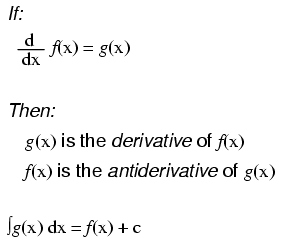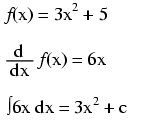# Learning Electronics

Learn to build electronic circuits

# The antiderivative (Indefinite integral)Notice something important here: taking the derivative of f(x) may precisely give you g(x), but taking the antiderivative of g(x) does not necessarily give you f(x) in its original form. Example:Note that the constant c is unknown! The original function f(x) could have been 3x2 + 5, 3x2 + 10, 3x2 + anything, and the derivative of f(x) would have still been 6x. Determining the antiderivative of a function, then, is a bit less certain than determining the derivative of a function.